Next: Hidden states Up: Hidden Markov models Previous: Hidden Markov models   Contents

## Markov chains

A Markov chain is a discrete stochastic process with discrete states and discrete transformations between them. At each time instant the system is in one of the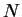possible states, numbered from one to. At regularly spaced discrete times, the system switches its state, possibly back to the same state. The initial state of the chain is denoted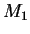and the states after each time of change are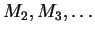. Standard first order Markov chain has the additional property that the probabilities of the future states depend only on the current state and not the ones before it . Formally this means that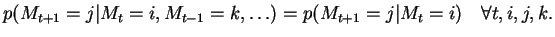(4.1)

This is called the Markov property of the chain.

Because of the Markov property, the complete probability distribution of the states of a Markov chain is defined by the initial distribution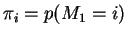and the state transition probability matrix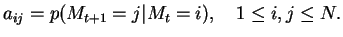(4.2)

Let us denote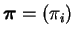and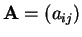. In the general case the transition probabilities could be time dependent, i.e.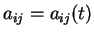, but in this thesis only the time independent case is considered.

This allows the evaluation of the probability of a sequence of states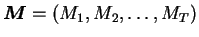, given the model parameters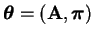, as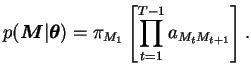(4.3)Next: Hidden states Up: Hidden Markov models Previous: Hidden Markov models   Contents
Antti Honkela 2001-05-30